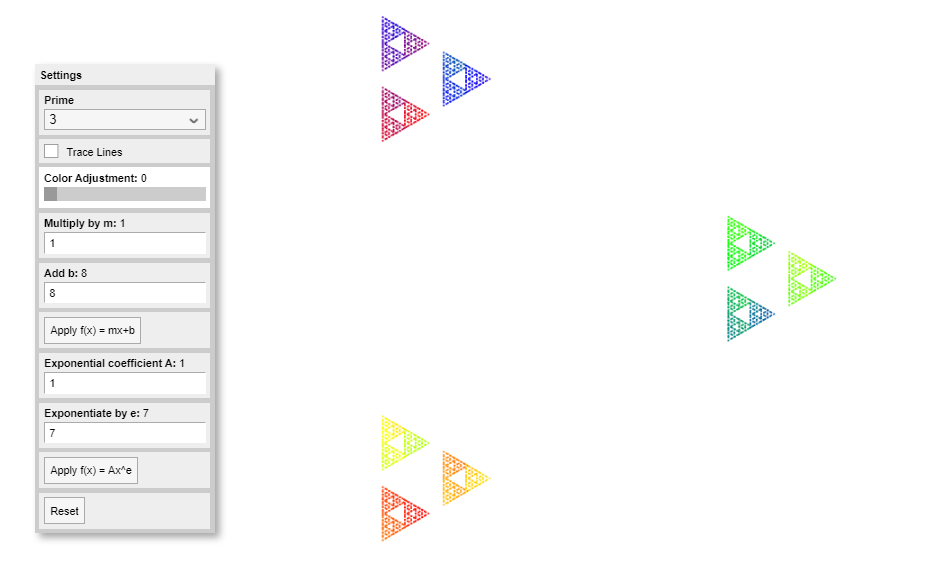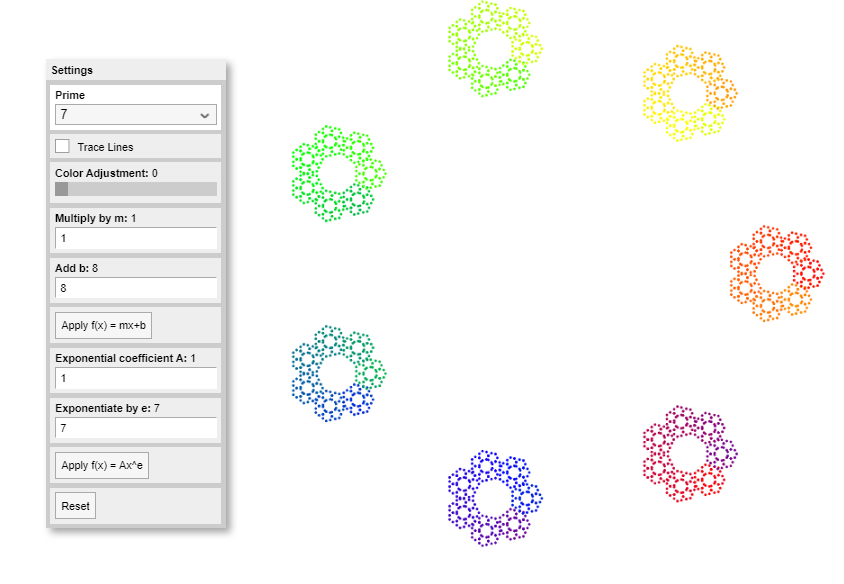Date started: November 2019
Leads: Gabriel Dorfsman-Hopkins, Edmund HarrisRoice Nelson, Kate Stange

###Abstract

The p-adic metric gives a way of measuring distance on the set of integers in a way that is reflects the multiplicative structure.  Here, smallness of a number depends on its divisibility by p, rather than its distance from zero. This metric allows for tools such as limits and analytic calculus to be used on integers in arithmetically meaningful ways, and gives rise to the p-adic numbers, which have become an invaluable tool in number theory.  Unfortunately, though the topology of the p-adics is well understood (they form a Cantor set), it is hard to come by models which accurately represent both their topology as well as the underlying addition and multiplication structure–compare this to the real numbers, where addition is translation and multiplication is scaling, both very easy to visualize in the standard number line.  The goal of this project is to fix this problem, that is, to create intuitive and interactive visual models of the p-adic numbers, where the topology and the structures of addition and multiplication are visible.

### Media

The following is a disk model of the 5-adic integers: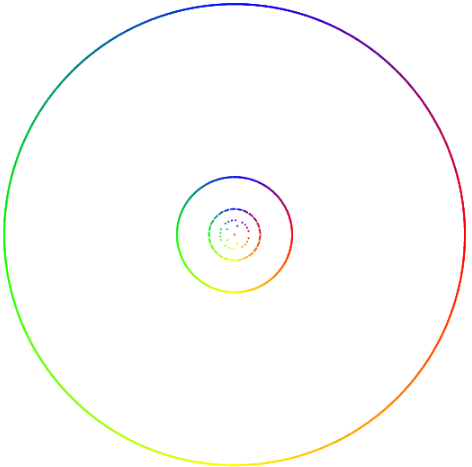Here 2 steps in the process of multiplication by 7: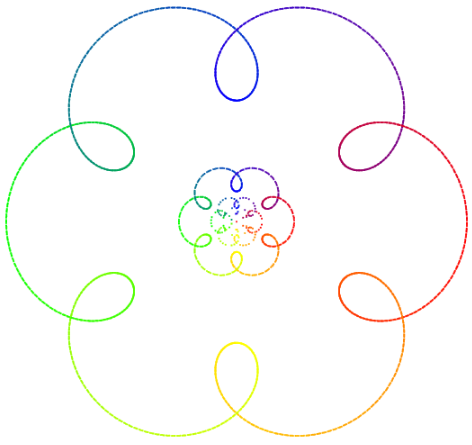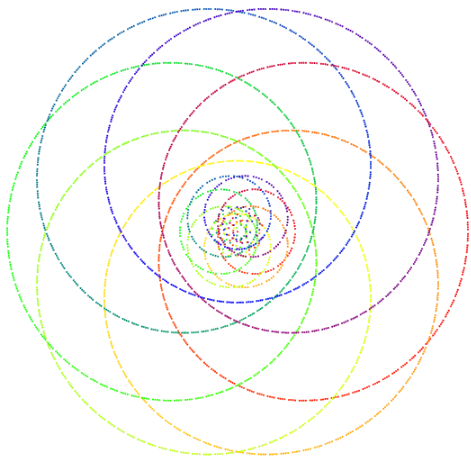Multiplication by 1 less than a power of 5 produces interesting patterns in this model.  Here are 124 and 624 respectively (multiplication by 5 is a contraction of the 5-adics, explaining the zoom between these two images).<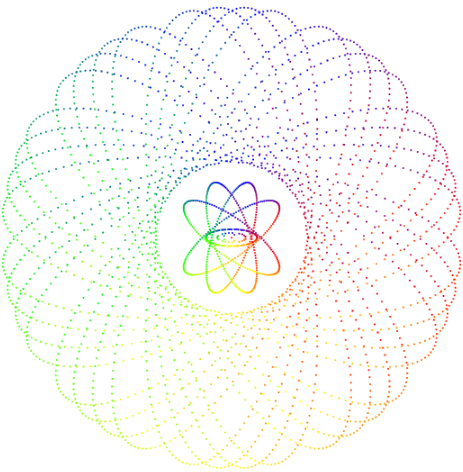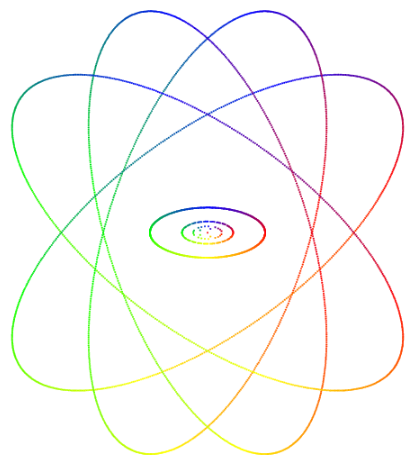This model also allows one to trace the points in transit: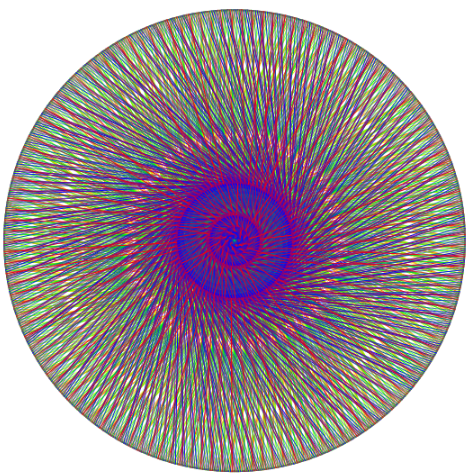Another model (due to Harris and Nelson) highlights the Cantor set topology more, and works well with additive structures (by doing nested rolls):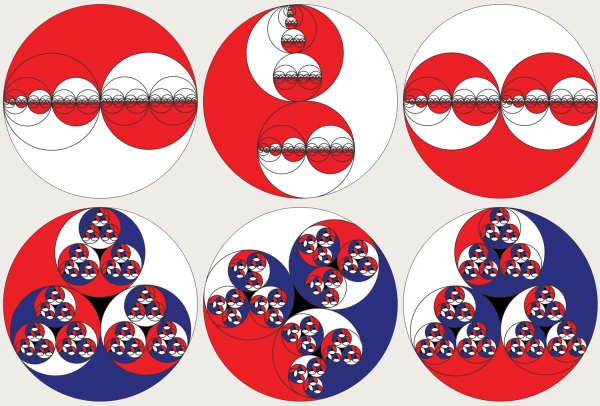Zooming in, here are the 2-adic,3-adic, and 5-adics in sequence.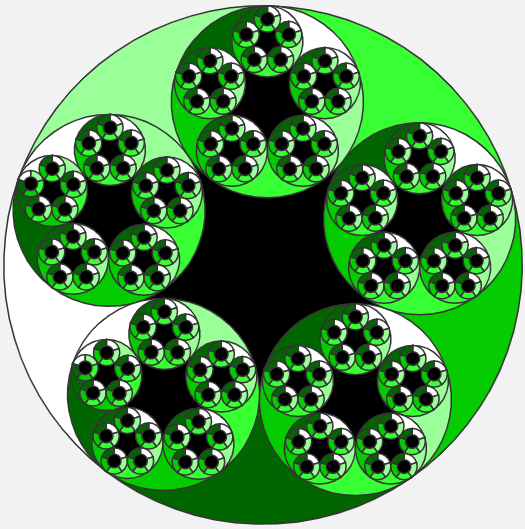A final model draws graphs of a function from Z/pnZ to itself, increasing n and trying to isolate the behavior which limits to the p-adics.More models are being discussed and constructed.